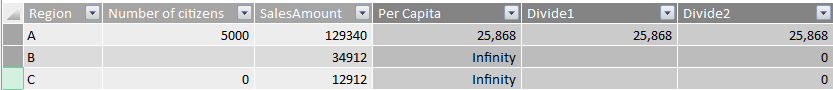# Power BI Pro Tip: DIVIDE() function

If you ever used Power Pivot to calculate things such as sales amount per capita or averages of some sort you will have run into the situation that the denominator (the column you want to divide by) is empty or zero. To cope with a potential division by zero, Power Pivot outputs Infinity. This can be seen in the screenshot below where the ‘Per Capita’ column is defined as:

`=[SalesAmount]/[Numer of citizens]`

Of course you can fix this by using

`IF(ISBLANK([InputColumn]))`

and others to work around the error. However, the DIVIDE() function makes this all a lot easier!

The DIVIDE() function takes two required and one optional parameters, which are: numerator, denominator and an optional value to return when division by zero occurs.

To see what DIVIDE() does, consider the following screenshot:The Divide1 column here is defined as:

`=DIVIDE([SalesAmount];[Number of citizens])`

Whereas the Divide2 column contains the following function:

`=DIVIDE([SalesAmount];[Number of citizens];0)`

The results are great, no Infinities are returned! By default DIVIDE() returns empty in case of a problem (Divide1 column). You can override this by specifying the third parameter so to return a fixed value in cased of a problem (Divide2 column in my example).

Hope this helps!